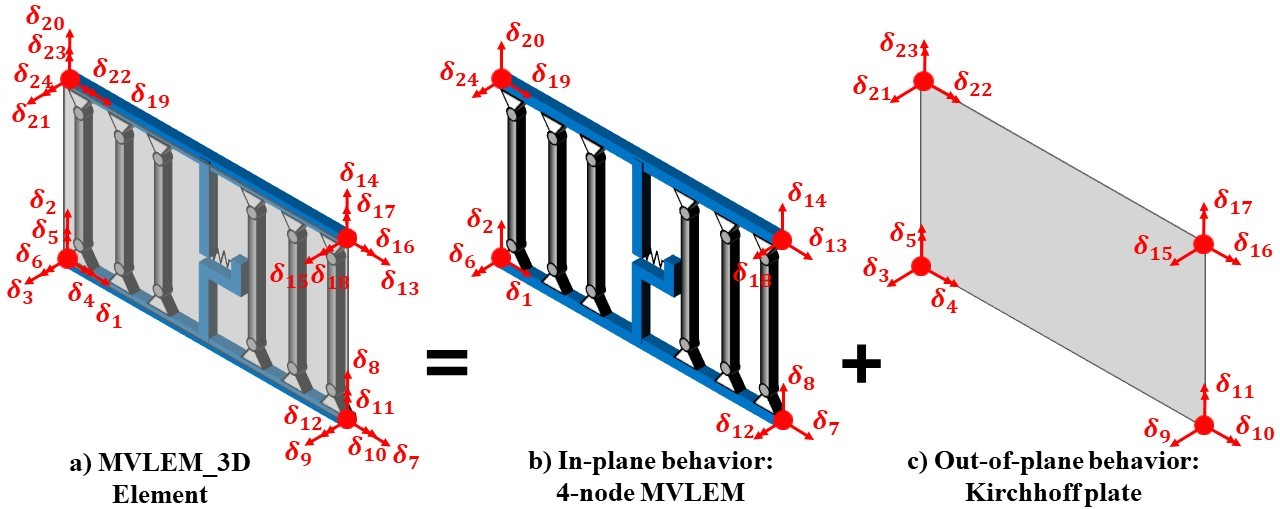# 4.2.7.11. MVLEM_3D - 3-D MVLEM Element for Flexure-Dominated RC Walls¶

Developed and implemented by:
Kristijan Kolozvari (CSU Fullerton)
Kamiar Kalbasi (CSU Fullerton)
Kutay Orakcal (Bogazici University)
John Wallace (UCLA)

The MVLEM_3D model (Figure 1a) is a three-dimensional four-node element with 24 DOFs for nonlinear analysis of flexure-controlled non-rectangular reinforced concrete walls subjected to multidirectional loading. The model is an extension of the two-dimensional, two-node Multiple-Vertical-Line-Element-Model (MVLEM). The baseline MVLEM, which is essentially a line element for rectangular walls subjected to in-plane loading, is extended to a three-dimensional model formulation by: 1) applying geometric transformation of the element in-plane degrees of freedom that convert it into a four-node element formulation (Figure 1b), as well as by incorporating linear elastic out-of-plane behavior based on the Kirchhoff plate theory (Figure 1c). The in-plane and the out-of-plane element behaviors are uncoupled in the present model.

This element shall be used in Domain defined with -ndm 3 -ndf 6.Figure 1: MVLEM_3D Element Formulation

element('MVLEM_3D', eleTag, *eleNodes, m, '-thick', *thick, '-width', *widths, '-rho', *rho, '-matConcrete', *matConcreteTags, '-matSteel', *matSteelTags, '-matShear', matShearTag, <'-CoR', c>, <'-ThickMod', tMod>, <'-Poisson', Nu>, <'-Density', Dens>)
 eleTag (int) unique element object tag eleNodes (list (int)) a list of four element nodes defined in the counter-clockwise direction m (int) number of element uniaxial fibers thick (list (float)) a list of m macro-fiber thicknesses widths (list (float)) a list of m macro-fiber widths rho (list (float)) a list of m reinforcing ratios corresponding to macro-fibers; for each fiber: $$rho_i = A_{s,i}/A_{gross,i} (1 < i < m)$$ matConcreteTags (list (int)) a list of m uniaxialMaterial tags for concrete matSteelTags (list (int)) a list of m uniaxialMaterial tags for steel matShearTag (int) tag of uniaxialMaterial for shear material c (float) location of center of rotation from the base (optional; default = 0.4 (recommended)) tMod (float) thickness multiplier (optional; default = 0.63 equivalent to 0.25Ig for out-of-plane bending) Nu (float) Poisson ratio for out-of-plane bending (optional; default = 0.25) Dens (float) density (optional; default = 0.0)